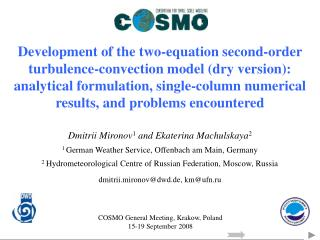DownloadDownload PresentationDmitrii Mironov 1 and Ekaterina Machulskaya 2

# Dmitrii Mironov 1 and Ekaterina Machulskaya 2

Download Presentation## Dmitrii Mironov 1 and Ekaterina Machulskaya 2

- - - - - - - - - - - - - - - - - - - - - - - - - - - E N D - - - - - - - - - - - - - - - - - - - - - - - - - - -
##### Presentation Transcript

1. Development of the two-equation second-order turbulence-convection model (dry version): analytical formulation, single-column numerical results, and problems encountered Dmitrii Mironov1 and Ekaterina Machulskaya2 1 German Weather Service, Offenbach am Main, Germany 2 Hydrometeorological Centre of Russian Federation, Moscow, Russia dmitrii.mironov@dwd.de, km@ufn.ru COSMO General Meeting, Krakow, Poland 15-19 September 2008

2. Recall … (UTCS PP Plan for 2007-2008) Task 1a: Goals, Key Issues, Expected Outcome Goals • Development and testing of a two-equation model of a temperature-stratified PBL • Comparison of two-equations (TKE+TPE) and one-equation (TKE only) models Key issues • Parameterisation of the pressure terms in the Reynolds-stress and the scalar-flux equations • Parameterisation of the third-order turbulent transport in the equations for the kinetic and potential energies of fluctuating motions • Realisability, stable performance of the two-equation model Expected outcome • Counter gradient heat flux in the mid-PBL • Improved representation of entrainment at the PBL top

3. One-Equation Model vs. Two-Equation Model – Key Differences Equation for <’2>, Production = Dissipation (implicit in all models that carry the TKE equations only). Equation for <w’’>, No counter-gradient term.

4. Convective Boundary Layer • Shear-free (zero geostrophic wind) and sheared (10 m/s geostrophic wind) • Domain size: 4000 m, vertical grid size: 1 m, time step: 1 s, simulation length: 4 h • Lower b.c. for : constant surface temperature (heat) flux of 0.24 K·m/s • Upper b.c. for  : constant temperature gradient of 3·10-3 K/m • Lower b.c. for U: no-slip, logarithmic resistance law to compute surface friction velocity • Upper b.c. for U: wind velocity is equal to geostrophic velocity • Initial temperature profile: height-constant temperature within a 780 m deep PBL, linear temperature profile aloft with the lapse rate of 3·10-3 K/m • Initial TKE profile: similarity relations in terms of z/h • Initial <’2> profile: zero throughout the domain • Turbulence moments are made dimensionless with the Deardorff (1970) convective velocity scales h, w*=(g<w’’>sfc)1/3 and * =<w’’>sfc/ w*

5. Mean Temperature in Shear-Free Convective PBL One-Equation and Two-Equation Models Red – one-equation model, green – two-equation model, blue – one-equation model with the Blackadar (1962) formulation for the turbulence length scale. Blackcurve shows the initial temperature profile.

6. Mean Temperature in Shear-Free Convective PBL (cont’d) One-Equation and Two-Equation Models vs. LES Data Potential temperature minus its minimum value within the PBL. Black dashed curve shows LES data (Mironov et al. 2000), red – one-equation model, green – two-equation model, blue – one-equation model with the Blackadar (1962) formulation for the turbulence length scale.

7. Potential-Temperature (Heat) Flux in Shear-Free Convective PBL One-Equation and Two-Equation Models vs. LES Data <w’’>made dimensionless with w**. Black dashed curve shows LES data, red – one-equation model, green – two-equation model, blue – one-equation model with the Blackadar formulation for the turbulence length scale.

8. Budget of Potential-Temperature Variance in Shear-Free Convective PBL One-Equation and Two-Equation Models vs. LES Data Solid curves – two-equation model, dashed curves – LES data. Red – mean-gradient production/destruction, green – third-order transport, blue – dissipation. The budget terms are made dimensionless with *2w*/h. Counter-gradient heat flux

9. Stably Stratified Boundary Layer • Wind forcing: 2 m/s geostrophic wind • Domain height: 2000 m, vertical grid size: 1 m, time step: 1 s, simulation length: 24 h • Lower b.c. for <’2>: (a) zero flux, <w’’2>sfc=0 K2·m/s, (b) non-zero flux, <w’’2>sfc=0.5 K2·m/s • Lower b.c. for : radiation-turbulent heat transport equilibrium, Tr4+Ts4+<w’’>sfc=0, logarithmic heat transfer law to compute the surface heat flux as function of the temperature difference between the surface and the first model level above the surface • Upper b.c. for : constant temperature gradient of 3·10-3 K/m • Lower b.c. for U: no-slip, logarithmic resistance law to compute surface friction velocity • Upper b.c. for U: wind velocity is equal to geostrophic velocity • Initial temperature profile: log-linear with 15 K temperature difference across a 200 m deep PBL, linear temperature profile aloft with the lapse rate of 3·10-3 K/m • Initial profiles of TKE and <’2>: similarity relations in terms of z/h

10. Effect of Horizontal Inhomogeneity of the Underlying Surfacewith Respect to the Temperature Equation for <’2>, Within the framework of two-equation model, <w’’2> is non-zero (transport of <’2> within the PBL) and may be non-zero at the surface (effect of horizontal inhmomogeneity) Within the framework of one-equation model, <w’’2> is entirely neglected

11. TKE and Potential-Temperature Variance in Stably Stratified PBL (strongly stable) Left panel – TKE, right panel – <’2>.Red – one-equation model, solid green – two-equation model, dashed green – two-equation model with non-zero <’2> flux.

12. Conclusions and Outlook • A dry version of a two-equation turbulence-convection model is developed and favourably tested through single-column numerical experiments • A number of problems with the new two-equation model have been encountered that require further consideration (sensitivity to the formulation of turbulence length/time scale, consistent formulation of “stability functions”, realisability) Ongoing and Future Work • Consolidation of a dry version of the two-equation model (c/o Ekaterina and Dmitrii), including further testing against LES data from stably stratified PBL (c/o Dmitrii in co-operation with NCAR) • Formulation and testing of a moist version of the new model

13. Thank you for your attention!